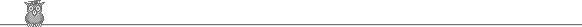### 13th Putnam 1953Problem A6

Show that √7, √(7 - √7), √(7 - √(7 + √7)), √(7 - √(7 + √(7 - √7))), ... converges and find its limit.

Solution

Let xn be the nth number in the sequence. We have xn+2 = √(7 - √(7 + xn)). Hence if xn < 2, then xn+2 > 2, and if xn > 2, then xn+2 < 2. So if the sequence converges, its limit must be 2.

If xn = 2 + ε, with 0 < ε < 1, then 9 + ε < 9 + ε + ε2/36 = (3 + ε/6)2, so xn+2 > √(7 - (3 + ε/6)) = √(4 - ε/6). But certainly ε/6 < ε/3 - ε2/144, so √(4 - ε/6) > 2 - ε/12. Thus xn+2 differs from 2 by less than ε/12. Similarly, if xn = 2 - ε, with 0 < ε < 1, then 9 - ε > 9 - ε/2 + ε2/144 = (3 - ε/12)2, so xn+2 < √(4 + ε/12) < 2 + ε/48.

So we have established that if |xn - 2| < 1, then |xn+2 - 2| < 1/12 |xn - 2|. But we certainly have |x1 - 2| < 1, and |x2 - 2| < 1, so xn converges to 2.